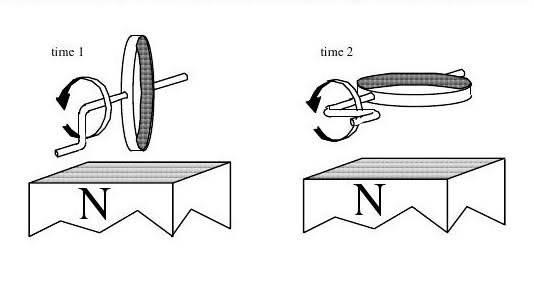# Direction of induced current in metal loop

## Homework Statement

A metal loop is attached to an axle with a handle as shown. The north pole of a magnet is placed below the loop and the handle turned so that the loop rotates counterclockwise at a constant angular speed.

On the two diagrams below, indicate the direction of the induced current in the loop at each of the instants shown. If the current is zero, state that explicitly. Explain how you determined your answers.none

## The Attempt at a Solution

On the first instant, I was thinking that maybe the induced current is zero because there's no flux through the loop? I'm completely not sure though. For the second instant, I'm pretty sure there is a current, but I don't know about the direction. Would it be clockwise around the loop? Since the current is trying to counteract the increasing flux? I don't know if that's true.

I am not confident in any of my answers, so any help is appreciated!

## Answers and Replies

Doc Al
Mentor
On the first instant, I was thinking that maybe the induced current is zero because there's no flux through the loop?
What determines the induced current? Is it simply the flux through the loop? Hint: Look up Faraday's law.

the magnetic field from the magnet generates an emf that determines the induced current. induced current produces a magnetic field opposing the change in flux. if flux is increasing in some direction, then the current will produce a magnetic field that decreases the flux in that direction, and if flux is decreasing in a direction, then the current will produce a B-field that increases the flux in that direction.

doesn't that mean there is no induced current in the first diagram? the magnetic field from the magnet does not flow through the cross section. the induced emf is proportional the the negative rate of change in magnetic flux.

Doc Al
Mentor
doesn't that mean there is no induced current in the first diagram?
No.
the magnetic field from the magnet does not flow through the cross section. the induced emf is proportional the the negative rate of change in magnetic flux.
What matters is the rate of change of the flux. How is the flux changing at the two points shown?

on the first diagram, the magnetic field and the plane of the loop are parallel to each other correct? so there must be zero flux.
on the second diagram, the plane of the loop and magnetic field are perpendicular to each other so flux is at its maximum.

is that right?

Doc Al
Mentor
on the first diagram, the magnetic field and the plane of the loop are parallel to each other correct? so there must be zero flux.
on the second diagram, the plane of the loop and magnetic field are perpendicular to each other so flux is at its maximum.

is that right?
That's correct. But what matters is not the flux at each point, but the rate at which the flux is changing.

Can you write an expression for the flux as a function of angle? (Just call the loop area A and the magnetic field B.) Then find its rate of change?

okay, so i have:
Φ= BAcosθ
dΦ/dθ = -BAsinθ

Doc Al
Mentor
okay, so i have:
Φ= BAcosθ
dΦ/dθ = -BAsinθ
Excellent. That's exactly what I wanted you to do.

So, at what angle is the rate of change--and thus the induced current--greatest? Where is it zero?

the angle is greatest at 90° since sin(90°) = 1. it is zero at 0°.

Doc Al
Mentor
the angle is greatest at 90° since sin(90°) = 1. it is zero at 0°.
Right. So how will you revise your thoughts about time 1 and time 2 in the diagram?

so, time 1 will have the greatest induced current, while time 2 will have the least induced current. therefore, will time 2 have zero current? would the direction of the current in time 1 be clockwise?

Doc Al
Mentor
so, time 1 will have the greatest induced current, while time 2 will have the least induced current. therefore, will time 2 have zero current?
Right.
would the direction of the current in time 1 be clockwise?
Viewed from the right, yes.

Ah, thank you Doc! that makes so much more sense now.

Doc Al
Mentor
My pleasure.